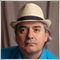10

hello.

is there a way to calculate RSI and Bollinger Bands from price (BID + ASK)/2 instead of BID price?

thanks5078

tapion: is there a way to calculate RSI and Bollinger Bands from price (BID + ASK)/2 instead of BID price?

Only Bid prices are recorded for OHLC bar rates. In MT5 (not MT4) however, there is also a record of the highest spread during the bar period, so you can use that in your calculations.

You would have to take the example code for the RSI indicator and change it to use "(Bid + MaxSpread/2)" instead, which approximates "(Bid+Ask)/2".

PS! In MT5, you can also theoretically build your own Bar rates that include individual Ask prices as well, since you have access to tick-data history.10

Fernando Carreiro:

Only Bid prices are recorded for OHLC bar rates. In MT5 (not MT4) however, there is also a record of the highest spread during the bar period, so you can use that in your calculations.

You would have to take the example code for the RSI indicator and change it to use "(Bid + MaxSpread/2)" instead, which approximates "(Bid+Ask)/2".

PS! In MT5, you can also theoretically build your own Bar rates that include individual Ask prices as well, since you have access to tick-data history.

do you mean that i have to modify the code of RSI indicator in mt5 ?

this is mql5 code, but i don't find where it refers to BID price, and i don't know where modify it with Bid + MaxSpread/2

#property copyright   "2009-2017, MetaQuotes Software Corp."
#property description "Relative Strength Index"
//--- indicator settings
#property indicator_separate_window
#property indicator_minimum 0
#property indicator_maximum 100
#property indicator_level1 30
#property indicator_level2 70
#property indicator_buffers 3
#property indicator_plots   1
#property indicator_type1   DRAW_LINE
#property indicator_color1  DodgerBlue
//--- input parameters
input int InpPeriodRSI=14; // Period
//--- indicator buffers
double    ExtRSIBuffer[];
double    ExtPosBuffer[];
double    ExtNegBuffer[];
//--- global variable
int       ExtPeriodRSI;
//+------------------------------------------------------------------+
//| Custom indicator initialization function                         |
//+------------------------------------------------------------------+
void OnInit()
{
//--- check for input
if(InpPeriodRSI<1)
{
ExtPeriodRSI=12;
Print("Incorrect value for input variable InpPeriodRSI =",InpPeriodRSI,
"Indicator will use value =",ExtPeriodRSI,"for calculations.");
}
else ExtPeriodRSI=InpPeriodRSI;
//--- indicator buffers mapping
SetIndexBuffer(0,ExtRSIBuffer,INDICATOR_DATA);
SetIndexBuffer(1,ExtPosBuffer,INDICATOR_CALCULATIONS);
SetIndexBuffer(2,ExtNegBuffer,INDICATOR_CALCULATIONS);
//--- set accuracy
IndicatorSetInteger(INDICATOR_DIGITS,2);
//--- sets first bar from what index will be drawn
PlotIndexSetInteger(0,PLOT_DRAW_BEGIN,ExtPeriodRSI);
//--- name for DataWindow and indicator subwindow label
IndicatorSetString(INDICATOR_SHORTNAME,"RSI("+string(ExtPeriodRSI)+")");
//--- initialization done
}
//+------------------------------------------------------------------+
//| Relative Strength Index                                          |
//+------------------------------------------------------------------+
int OnCalculate(const int rates_total,
const int prev_calculated,
const int begin,
const double &price[])
{
int    i;
double diff;
//--- check for rates count
if(rates_total<=ExtPeriodRSI)
return(0);
//--- preliminary calculations
int pos=prev_calculated-1;
if(pos<=ExtPeriodRSI)
{
//--- first RSIPeriod values of the indicator are not calculated
ExtRSIBuffer=0.0;
ExtPosBuffer=0.0;
ExtNegBuffer=0.0;
double SumP=0.0;
double SumN=0.0;
for(i=1;i<=ExtPeriodRSI;i++)
{
ExtRSIBuffer[i]=0.0;
ExtPosBuffer[i]=0.0;
ExtNegBuffer[i]=0.0;
diff=price[i]-price[i-1];
SumP+=(diff>0?diff:0);
SumN+=(diff<0?-diff:0);
}
//--- calculate first visible value
ExtPosBuffer[ExtPeriodRSI]=SumP/ExtPeriodRSI;
ExtNegBuffer[ExtPeriodRSI]=SumN/ExtPeriodRSI;
if(ExtNegBuffer[ExtPeriodRSI]!=0.0)
ExtRSIBuffer[ExtPeriodRSI]=100.0-(100.0/(1.0+ExtPosBuffer[ExtPeriodRSI]/ExtNegBuffer[ExtPeriodRSI]));
else
{
if(ExtPosBuffer[ExtPeriodRSI]!=0.0)
ExtRSIBuffer[ExtPeriodRSI]=100.0;
else
ExtRSIBuffer[ExtPeriodRSI]=50.0;
}
//--- prepare the position value for main calculation
pos=ExtPeriodRSI+1;
}
//--- the main loop of calculations
for(i=pos;i<rates_total && !IsStopped();i++)
{
diff=price[i]-price[i-1];
ExtPosBuffer[i]=(ExtPosBuffer[i-1]*(ExtPeriodRSI-1)+(diff>0.0?diff:0.0))/ExtPeriodRSI;
ExtNegBuffer[i]=(ExtNegBuffer[i-1]*(ExtPeriodRSI-1)+(diff<0.0?-diff:0.0))/ExtPeriodRSI;
if(ExtNegBuffer[i]!=0.0)
ExtRSIBuffer[i]=100.0-100.0/(1+ExtPosBuffer[i]/ExtNegBuffer[i]);
else
{
if(ExtPosBuffer[i]!=0.0)
ExtRSIBuffer[i]=100.0;
else
ExtRSIBuffer[i]=50.0;
}
}
//--- OnCalculate done. Return new prev_calculated.
return(rates_total);
}
//+------------------------------------------------------------------+• www.mql5.com
Choose a suitable trading strategy and subscribe to it with a few clicks. All Signals are provided with detailed statistics and informative charts. Become a trading signal provider and sell subscriptions to thousands of traders around the world. With the Signals service, your successful strategy can generate income with a small start-up budget...5078

tapion:

do you mean that i have to modify the code of RSI indicator in mt5 ?

this is mql5 code, but i don't find where it refers to BID price, and i don't know where modify it with Bid + MaxSpread/2

1. Yes, you have to modify the code of the RSI indicator.
2. No, you will not find a reference to "BID" because all prices are already based on "bid".
3. The code will have to be modified to use the second, more verbose, form of the OnCalculate() event handler which includes the spread data:
```int OnCalculate (const int rates_total,      // size of input time series
const int prev_calculated,  // bars handled in previous call
const datetime& time[],     // Time
const double& open[],       // Open
const double& high[],       // High
const double& low[],        // Low
const double& close[],      // Close
const long& tick_volume[],  // Tick Volume
const long& volume[],       // Real Volume